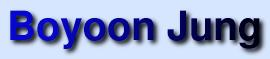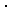Boyoon Jung's Library Manual

bj::MultivariateGaussian Class Reference [Machine Learning Techniques]

A multivariate gaussian distribution. More...

#include <gaussian.h>

List of all members.

Public Member Functions

MultivariateGaussian (int dimension, gsl_vector *mean=0, gsl_matrix *cov=0)
A constructor.
~MultivariateGaussian (void)
A destructor.
double p (gsl_vector *x, gsl_vector *mean, gsl_matrix *cov)
Compute the probability of a data point x with given mean and covariance.
double p (gsl_vector *x)
Compute the probability of a data point x.
gsl_vector * mean (void)
Get the current mean vector.
void setMean (gsl_vector *m)
Set a mean vector.
gsl_matrix * covariance (void)
Get the current covariance matrix.
void setCovariance (gsl_matrix *cov)
Set a covariance matrix.

Detailed Description

A multivariate gaussian distribution.

Constructor & Destructor Documentation

 bj::MultivariateGaussian::MultivariateGaussian ( int dimension, gsl_vector * mean = 0, gsl_matrix * cov = 0 )

A constructor.

Parameters:
 dimension The dimension of data points. mean A mean vector. cov A covariance matrix.

 bj::MultivariateGaussian::~MultivariateGaussian ( void )
 A destructor.

Member Function Documentation

 gsl_matrix* bj::MultivariateGaussian::covariance ( void ) [inline]
 Get the current covariance matrix.

 gsl_vector* bj::MultivariateGaussian::mean ( void ) [inline]
 Get the current mean vector.

 double bj::MultivariateGaussian::p ( gsl_vector * x )
 Compute the probability of a data point x.

 double bj::MultivariateGaussian::p ( gsl_vector * x, gsl_vector * mean, gsl_matrix * cov )
 Compute the probability of a data point x with given mean and covariance.

 void bj::MultivariateGaussian::setCovariance ( gsl_matrix * cov )
 Set a covariance matrix.

 void bj::MultivariateGaussian::setMean ( gsl_vector * m ) [inline]
 Set a mean vector.

The documentation for this class was generated from the following file:
ResearchProjectsPublications

ToolsBjLib ManualPlayer/StagePyPlayerMicroStrain IMUGPS/DGPSParticle Filter

PersonalWelcomeCurriculum VitaeResumeContactUSCUSC CS DeptCRESRobotics LabRESL